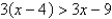Try out our new practice tests completely free!# Algebra and Trigonometry

Mathematics

## Quiz 2 : Solving Equations and InequalitiesStudy FlashcardsLooking for Calculus Homework Help?

## Quiz 2 :Solving Equations and InequalitiesSolve the following equation.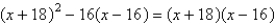Free
Multiple Choice

A

Tags
Choose question tagSet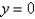and solve the resulting equation.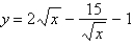Free
Multiple Choice

E

Tags
Choose question tagSolve: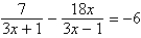Free
Multiple Choice

B

Tags
Choose question tagSimplify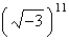and write the answer in standard form.
Multiple Choice
Tags
Choose question tagSolve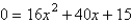using the quadratic formula.
Multiple Choice
Tags
Choose question tagSolve the following quadratic equation by factoring.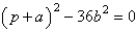Multiple Choice
Tags
Choose question tagWhich of the following is a zero of the given function?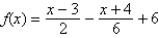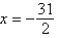,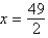'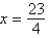,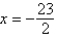Multiple Choice
Tags
Choose question tagSolve the following equation using any convenient method.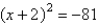Multiple Choice
Tags
Choose question tagSimplify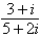and write the answer in standard form.
Multiple Choice
Tags
Choose question tagSimplify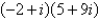and write the answer in standard form.
Multiple Choice
Tags
Choose question tagFind the x-intercepts of the graph of the equation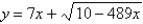Multiple Choice
Tags
Choose question tagWhich of the following is a zero of the given function?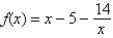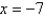,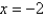,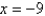,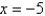Multiple Choice
Tags
Choose question tagSolve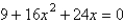by factoring.
Multiple Choice
Tags
Choose question tagDetermine any point(s) of intersection between the following equations.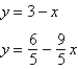Multiple Choice
Tags
Choose question tagSolve the following equation.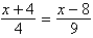Multiple Choice
Tags
Choose question tagFind all solutions of the following equation algebraically.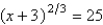Multiple Choice
Tags
Choose question tagFind all solutions of the following equation algebraically.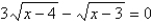Multiple Choice
Tags
Choose question tagFind all solutions of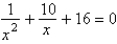.
Multiple Choice
Tags
Choose question tagSolve: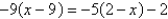Multiple ChoiceSolve: